# Battery ESS (Generic)

Description of the Battery ESS (Generic) component in Schematic Editor

The Battery ESS (Generic) component, shown in Table 1, is a Schematic Editor library block from the Distributed Energy Resources section, of the Microgrid library. It is capable of operating in isochronous, droop, and grid following mode.

Table 1. Battery ESS (Generic) component in the HIL Toolbox
component component dialog window component parameters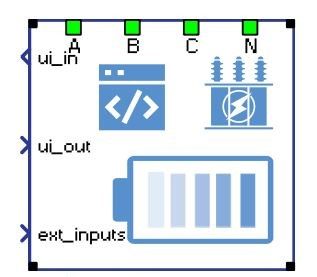• Property tabs:
• 1 - General
• 2 - Battery
• 3 - Grid codes
• 4 - Conv. Extras
• 5 - Grid Extras

## Battery ESS (Generic) block diagram

The component consists of two main parts: a high level control subsystem and a low level control subsystem with a power stage and necessary measurements included.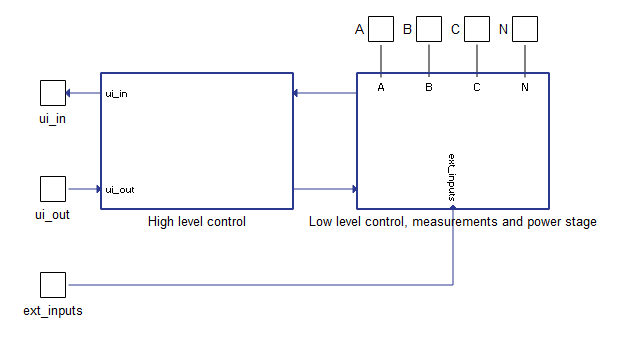Figure 1. Battery ESS (Generic) block diagram
High level control contains:
• PQ Control block - Contains regulators for active and reactive power control. The PQ Control block receives power references and measured powers after the transformer (grid side) and calculates refererence output currents. These current references are fed to the low level control logic. This control block is active in the grid following mode of operation.
• Ramping elements - Limit the ROC (rate of change) of the active power reference, reactive power reference, voltage reference, and frequency reference. The rate of change for each function is configured through a corresponding input signal.
• Frequency droop functionality - Calculates frequency reference signal when droop control mode is activated. This control unit is described in the documentation for the Frequency droop unit in DER generic components.
• Voltage droop functionality - Calculates voltage references signal when droop control mode is activated. This control unit is described in Voltage droop unit in DER generic components.
Low level control subsystem main parts:
• Currents and Voltage/Frequency control block - Contains regulators for inner current and voltage control loops. Input signals to the Currents Control block are reference currents and measured currents before the transformer (converter side). The input signal to the Voltage Control block is the reference voltage and measured voltage before the transformer (converter side).
• Fault state machine - Checks all measurements, signals the fault state, and sends an alarm message. It also immediately opens the MCB (main circuit breaker) on any detected fault. A list of implemented faults is shown in Faults/Alarm messages.

## Battery ESS (Generic) power stage

The power stage is shown in Figure 2. The main elements include the average model of the inverter, the input L filter, the transformer, and the MCB that connects the plant to the grid. The transformer can be excluded from the power stage if it is not necessary. Power stage elements are parametrized from the component dialog box.

## Battery ESS (Generic) inputs and outputs

This component has one user interface input, one user interface output, and one external input. The user interface input and user interface output are each arrays of 50 elements, while the external input is an array of 5 elements. Table 2 describes the input signals and their order, while Table 3 describes the output signals and their order. Lastly, Table 4 describe the external battery energy storage input signals and their order.

All these signals are also defined in the corresponding Battery ESS (Generic) UI subsystem with built-in Probe components (for output signals) and SCADA Input components (for input signals), as shown in Table 5. These signals were split and joined by a properly configured DER (Generic) Output Split and DER (Generic) Control Join components. The Battery ESS (Generic) UI subsystem is located in the same place in the Schematic Editor library as the Battery ESS (Generic) component, and it can be unlinked from the library and adopted to the specific needs. Unit [pu] is associated to nominal values, which are either inserted as general tab parameters or derived from them.

Table 2. Battery ESS (Generic) user interface inputs
Number Input Description Signal range Default value
0 Enable A digital input that enables the converter. The converter is enabled when the input value is high. 0 or 1 0
1 Converter mode An analog input that sets the operation mode of the converter. Available modes and their respective codes are:
• grid following mode (code 0)
• droop (code 1)
• isochronous (code 2)
0 or 1 or 2 0
2 Reset alarms A digital input that resets alarms if the converter is in a fault 0 or 1 0
3 Pref An analog input that is the active power reference. This reference is active in grid following and droop mode. [pu] 0
4 Qref An analog input that sets the reactive power reference. This reference is active in grid following and droop mode. [pu] 0
5 Frequency droop offset An analog input that sets the offset for frequency in frequency droop control mode. [pu] 0
6 Frequency droop coeff An analog input that sets the frequency droop coefficient for the frequency droop control mode [%] [0, 100] 2
7 Voltage droop offset An analog input that sets the voltage droop coefficient for the voltage droop control mode [%] [0, 100] 4
8 Vrms_ref An analog input that sets the voltage reference. This reference is active in isochronous mode of operation. [pu] > 0 1
9 Fref An analog input that sets the frequency reference. This reference is active in the isochronous mode of operation. [pu] > 0 1
10 Max SOC An analog input that sets the maximum allowed state of charge of the battery. [%] [55.0, 95.0] 90
11 Min SOC An analog input that sets the minimum allowed state of charge of the battery. [%] [5.0, 45.0] 10
12 Pref rate of change An analog input that sets the rate of change of the active power reference ramping. [pu/s] >= 0.001 0.1
13 Qref rate of change An analog input that sets the rate of change of the reactive power reference ramping. [pu/s] >= 0.001 0.1
14 Vrms_ref rate of change An analog input that sets the rate of change of the voltage reference ramping. [pu/s] >= 0.001 0.1
15 Fref rate of change An analog input that sets the rate of change of the frequency reference ramping. [pu/s] >= 0.0001 0.02
16 LVRT enable Digital input that enables the LVRT unit. The LVRT unit is enabled when the input value is high. 0 or 1 0
17 LVRT Q contribution An analog input that specifies additional reactive power contribution per percent of voltage drop in the case of a LVRT event. [%] >= 0 2
18 LVRT P set An analog input that defines the active power reference in the case of a LVRT event. [pu] >= 0 1
19 V alarm upper limit* Upper limit for the Grid voltage out of range error [pu] > 1 1.5
20 V alarm lower limit* Lower limit for the Grid voltage out of range error [pu] [0, 1) 0.5
21 F alarm upper limit* Upper limit for the Grid frequency out of range error [pu] > 1 1.5
22 F alarm lower limit* Lower limit for the Grid frequency out of range error [pu] [0, 1) 0.5
23 S alarm upper limit* Upper limit for the Over power protection [pu] > 1 1.5
24 I alarm upper limit* Upper limit for the Over current protection [pu] > 1 1.5
25 VoltVAr enable Digital input that enables the VoltVAr unit. The VoltVAr unit is enabled when the input value is high. 0 or 1 0
26 HzWatt enable Digital input that enables the HzWatt unit. The HzWatt unit is enabled when the input value is high. 0 or 1 0
27 VoltWatt enable Digital input that enables the VoltWatt unit. The VoltWatt unit is enabled when the input value is high. 0 or 1 0
28-49 reserved_ins Inputs that are not used. 0

* If the values for inputs 19 to 24 are all zeroes, default values will be used.

Table 3. Battery ESS (Generic) user interface outputs
Number Output Description
0 Enable_fb A digital output describing the converter enable/disable state. The converter is enabled if the output is high.
1 Converter_mode_fb An analog output describing which control mode the converter operates in.
2 Pref_fb_kW An analog output with the instantaneous value of the applied power reference in the converter. [kW]
3 Qref_fb_kVAr An analog output with the instantaneous value of the applied reactive power reference in the converter. [kVAr]
4 Pnom_kW An analog output with the value of nominal active power of the converter. [kW]
5 Qnom_kVAr An analog output with the value of nominal reactive power of the converter. [kVAr]
6 Snom_kVA An analog output with the value of nominal apparent power of the converter. [kVA]
7 Vnom_LL_V An analog output with the value of nominal converter's voltage. [V]
8 Fnom_Hz An analog output with the value of nominal converter's frequency. [Hz]
9 Vrms_ref_fb_V An analog output with feedback information about the RMS value of the reference voltage. [V]
10 Fref_fb_Hz An analog output with feedback information about the reference frequency value. [Hz]
11 Vgrid_rms_meas_kV An analog output reporting the RMS value of the grid voltage, measured at the grid side of the transformer. [kV]
12 Fmeas_Hz An analog output reporting the value of the grid voltage frequency. [Hz]
13 Pmeas_kW An analog output reporting the instantaneous value of the three-phase active power output of the converter. [kW]
14 Pa_meas_kW An analog output reporting the instantaneous value of the phase A active power output of the converter. [kW]
15 Pb_meas_kW An analog output reporting the instantaneous value of the phase B active power output of the converter. [kW]
16 Pc_meas_kW An analog output reporting the instantaneous value of the phase C active power output of the converter. [kW]
17 Qmeas_kVAr An analog output reporting the instantaneous value of the three-phase reactive power output of the converter. [kVAr]
18 Qa_meas_kVAr An analog output reporting the instantaneous value of the phase A reactive power output of the converter. [kVAr]
19 Qb_meas_kVAr An analog output reporting the instantaneous value of the phase B reactive power output of the converter. [kVAr]
20 Qc_meas_kVAr An analog output reporting the instantaneous value of the phase C reactive power output of the converter. [kVAr]
21 Smeas_kVA An analog output reporting the instantaneous value of the three-phase apparent power output of the converter. [kVA]
22 PFmeas An analog output reporting the value of the converter output power factor.
23 Vconv_rms_meas_V An analog output reporting the RMS value of the converter voltage, measured at the converter side of the transformer. [V]
24 SOC An analog output reporting the state of charge value of the battery. [%]
25 SOH An analog output reporting the state of health value of the battery. [%]
Note: This functionality is not currently supported. Value is set to 0.
26 Vbatt_V An analog output reporting the instantaneous value of DC voltage at the output of the battery. [V]
27 Ibatt_A An analog output reporting the instantaneous value of DC current at the output of the battery. [A]
28 MCB_status A digital output reporting the information about the state of the main circuit breaker (contactor). The contactor is closed if the value is high.
29 state An analog output reporting the code of the state of the converter.
30 alarm_msg An analog output reporting the value of the alarm message. In order for the converter to return to normal operation after the alarm has been triggered, all alarms must be reset.
31 Batt_cap_nom_Ah An analog output reporting the value of the nominal battery capacity. [Ah]
32 Lvrt_active_status A digital output that signals the status of the LVRT unit. If high, the LVRT unit is active and contributing active and reactive power to the grid, otherwise it is not active.
33-49 reserved_outs Outputs that are not used.
Table 4. Battery ESS (Generic) external inputs
Number Input Description Signal range
0 Initial SOC Defines initial state of charge of the battery at simulation start. [%] [0, 100]
1 Initial SOH Defines initial state of health of the battery at simulation start. This value reduces nominal battery capacity. [%] (0, 100]
Note: In order preserve backward compatibility, 0 values are internally substituted with 100.
2-4 reserved_exts Inputs that are not used.

## Battery ESS (Generic) operational states

Table 6. Main state machine states
Code Decription
1 Starting up state – Represents the state of the converter between the moment signal activation is triggered and the moment when the converter starts operating, e.g. system checking and synchronization time.
2 Running state - Represents the operational state of the converter.
3 Disabled state - Reports if the converter is not enabled/operational.
4 Fault state – Represents the state of the converter if it encounters a fault. It is necessary to reset the alarms once the converter enters this state.
Table 7. Faults/Alarm messages
Code Description
0 None of the alarms have been triggered
1 Over current protection. Input phase currents are greater than the maximum specified value.
2 Grid voltage out of range. Grid voltage is outside the specified range. This protection is active in grid following mode. If the voltage restores, the fault will be automatically reset.
3 Grid frequency out of range. Grid frequency is outside the specified range. This protection is active in grid following mode. If the frequency restores, the fault will be automatically reset.
4 Over power protection. Measured apparent power is greater than the maximum allowed apparent power.
5 SOC at critical level. If the SOC is higher than absolute maximum value or lower than the absolute minimum value of SOC.

## Component dialogue box and parameters

The Battery ESS (Generic) component dialogue box consists of four tabs for specifying parameters of the component. Unit [pu] is associated to nominal values, which are inserted as general tab parameters or derived from them.

## Tab: "1 - General"

In this component tab, the user can specify general parameters of the Battery ESS and the grid.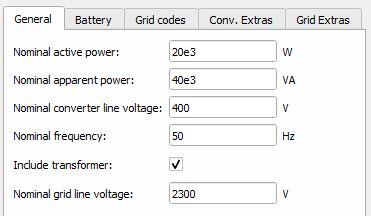Figure 5. Battery ESS (Generic) "1 - General" parameters.
Table 8. Battery ESS (Generic) "1- General" parametrs description
Parameter Code name Description
Nominal active power Pnom Battery ESS nominal active power. [W]
Nominal apparent power Snom Battery ESS nominal apparent power. [VA]
Nominal converter line voltage Vnom_LL Battery ESS nominal line voltage (transformer's primary side). [V]
Nominal frequency fnom Battery ESS Nominal frequency. [Hz]
Include transformer include_transformer Include the transformer in the Battery ESS power stage model.
Nominal grid line voltage Vnom_sec_LL Nominal line voltage of the grid (transformer's secondary side). [V]

## Tab: "2 - Battery"

In this component tab, parameters that are related to the Battery system part of the converter can be specified.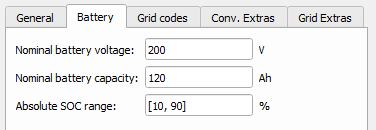Figure 6. Batery ESS (Generic) "2 - Battery" parameters.
Table 9. Batery ESS (Generic) "2 - Battery" parameters description
Parameter Code name Description
Nominal battery voltage nom_batt_voltage Nominal battery voltage. [V]
Nominal battery capacity nom_batt_capacity Nominal battery capacity. [Ah]
Absolute SOC range soc_range Absolute allowed SOC range. [%]

## Tab: "3 - Grid codes"

In this component tab, different Grid code parameters can be specified. Currently, LVRT (Low Voltage Ride Through), VoltVAr, HzWatt, and VoltWatt functionalities are supported.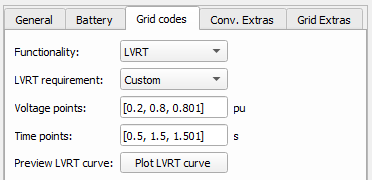Figure 7. Battery ESS (Generic) "3 - Grid codes" LVRT parameters
Table 10. Battery ESS (Generic) "3 - Grid codes" LVRT parameters
Parameter Code name Description
LVRT requirement lvrt_req Automatically parametrizes voltage and time points according to the chosen requirements. If the Custom option is selected, voltage and current points become available for editing.
Voltage points vs_lvrt LVRT curve voltage points. [pu]
Time points ts_lvrt LVRT curve time points. [s]
Preview LVRT curve preview Displays the created LVRT curve.

Implementation details for the LVRT unit are given on the LVRT in generic DER components page.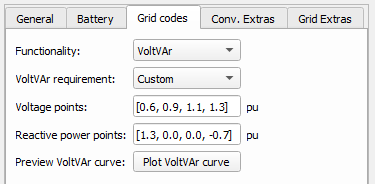Figure 8. Battery ESS (Generic) "3 - Grid codes" VoltVAr parameters
Table 11. Battery ESS (Generic) "3 - Grid codes" VoltVAr parameters
Parameter Code name Description
VoltVAr requirement voltvar_req Allows for defining voltage and reactive power points. Currently, only the Custom option is supported.
Voltage points vs_voltvar VoltVAr curve voltage points.
Reactive power points qs_voltvar VoltVAr curve reactive power points.
Preview VoltVAr curve preview_voltvar Displays the created VoltVAr curve.

If LVRT and VoltVAr functionalities are both enabled, then the VoltVAr curve has priority over the LVRT reactive power contribution setting (Q_contribution).

The VoltVAr curve is linearly extrapolated for voltage values below and above the minimum and maximum values. Also, the curve is limited by a maximum value of reactive power which can be produced or stored. This limit is calculated as , where opc_pu is the overpower capability.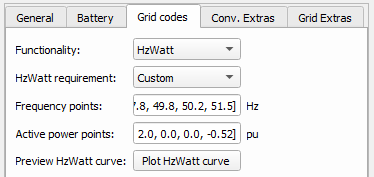Figure 9. Battery ESS (Generic) "3 - Grid codes" HzWatt parameters
Table 12. Battery ESS (Generic) "3 - Grid codes" HzWatt parameters
Parameter Code name Description
HzWatt requirement hzwatt_req Allows for defining frequency and active power points. Currently, only the Custom option is supported.
Frequency points fs_hzwatt HzWatt curve frequency points.
Active power points ps_hzwatt HzWatt curve active power points.
Preview HzWatt curve preview_hzwatt Displays the created HzWatt curve.

If LVRT and HzWatt functionalities are both enabled, then the HzWatt curve has priority over the LVRT active power contribution setting.

If the frequency value is below the minimum value or above the maximum value, then the Grid frequency out of range error will appear. The HzWatt curve is limited by a maximum value of active power which can be produced or stored. This limit is calculated as , where opc_pu is the overpower capability.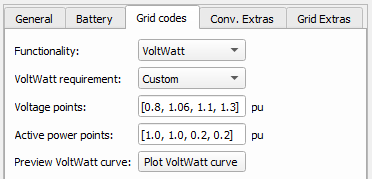Figure 10. Battery ESS (Generic) "3 - Grid codes" VoltWatt parameters
Table 13. Battery ESS (Generic) "3 - Grid codes" VoltWatt parameters
Parameter Code name Description
VoltWatt requirement voltwatt_req Allows for defining voltage and active power points. Currently, only the Custom option is supported.
Voltage points vs_voltwatt VoltWatt curve voltage points.
Active power points ps_voltwatt VoltWatt curve active power points.
Preview VoltWatt curve preview_voltwatt Displays the created VoltWatt curve.

If LVRT and VoltWatt functionalities are both enabled, then the VoltWatt curve has priority over the LVRT active power contribution setting. But, if HzWatt and VoltWatt functionalities are both enabled, then the HzWatt curve has priority over the VoltWatt curve.

The VoltWatt curve is linearly extrapolated for voltage values below and above the minimum and maximum values. Also, the curve is limited by a maximum value of active power which can be produced or stored. This limit is calculated as , where opc_pu is the overpower capability.

## Tab: "4 - Conv. Extras"

In this component tab, additional parameters that are related to the converter can be specified.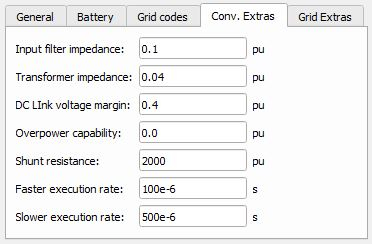Figure 11. Batery ESS (Generic) "4 - Conv. Extras" parameters.
Table 14. Batery ESS (Generic) "4 - Conv. Extras" parameters description
Parameter Code name Description
Input filter impedance wL_pu Input filter impedance. Input filter is a simple L type filter. See the Figure 2. [pu]
Transformer impedance Zt_n Determines the transformer impedance. The position of this transformer is shown in Figure 2. [pu]
DC link voltage margin dc_link_margin Voltage margin over the minimal allowed DC link voltage. This value restricts the maximum voltage that a converter can generate. [pu]
Overpower capability opc_pu Determines the overpower capability of the converter. [pu]
Shunt resistance Rshunt_pu The value of the shunt resistances that are used for the measurement of phase voltages at the converter input. The position of shunts is shown in Figure 2. [pu]
Faster execution rate Tfast The Faster execution rate at which part of the inner signal processing of the component will be executed. Should be approximately 5 to 10 times faster than the Slower execution rate. [s]
Slower execution rate Tslow The Slower execution rate, at which part of the inner signal processing of the component will be executed. This execution rate is inherited by the connected UI subsystem. Should be approximately 5 to 10 times slower than the Faster execution rate. [s]

## Tab: "5 - Grid Extras"

In this component tab, additional parameters that are related to the grid can be specified.Figure 12. Batery ESS (Generic) "5 - Grid Extras" parameters.
Table 15. Batery ESS (Generic) "5 - Grid Extras" parameters description
Parameter Code name Description
Grid Short Circuit power Sg_sc_pu Short circuit apparent power of the grid that the converter is connected to. This parameter doesn't influence the power stage of the model, only the control part. Stable operation of the converter can be achived by tuning this parameter. [pu]
Grid inductive power factor PFg Power factor of the grid that the converter is connected to. This power factor occurs when the grid is short-circuited. This parameter doesn't influence the power stage of the model, only the control part. Stable operation of the converter can be achieved by adjusting this parameter. [pu]

## Example

Overall behavior and control methodologies can be better understood with the use of the given example:

Model name: simple_battery_ess_gen.tse